# ML_Unit_2_Part_A

Education
May. 8, 2021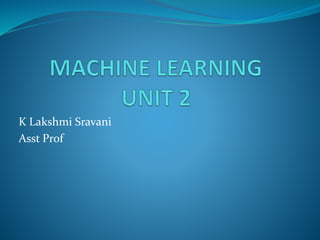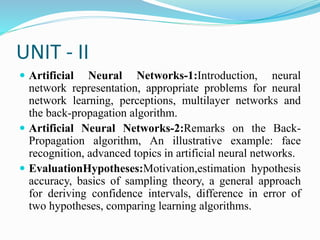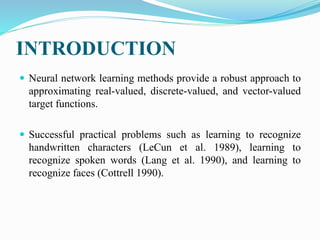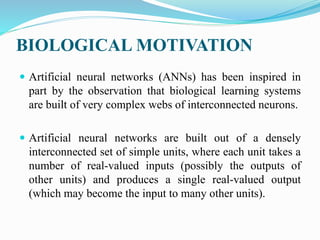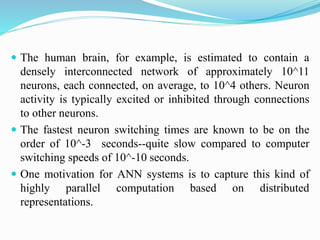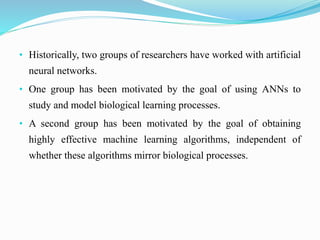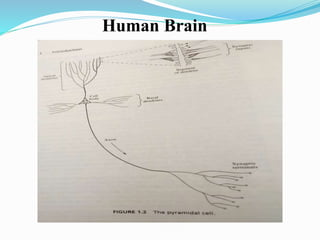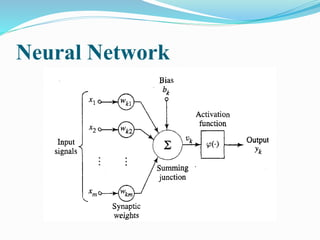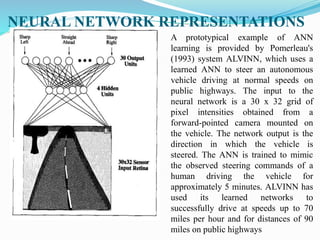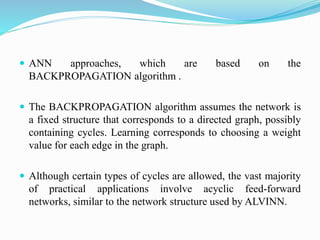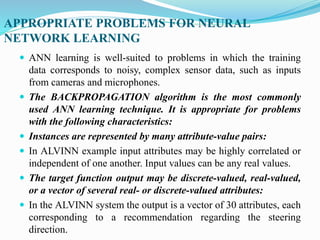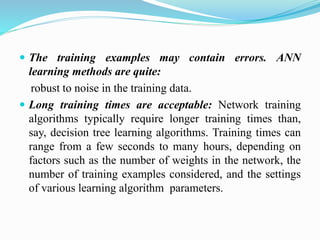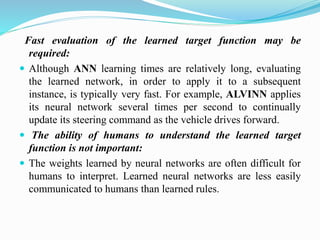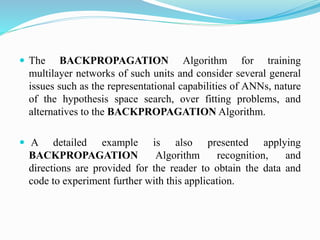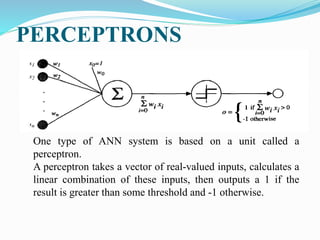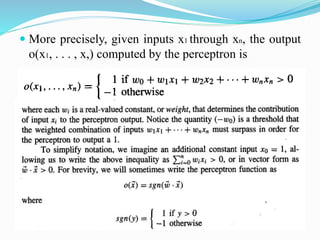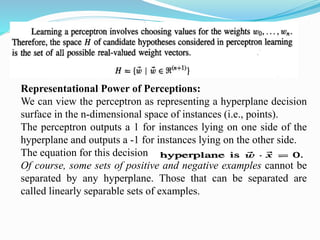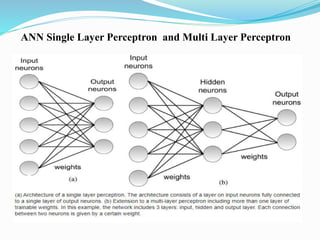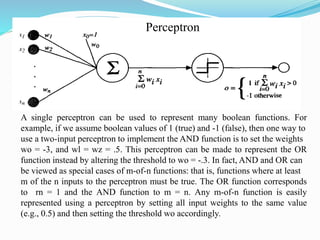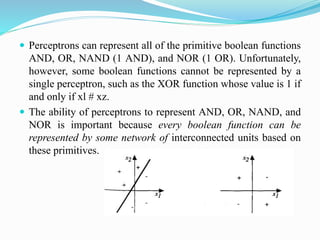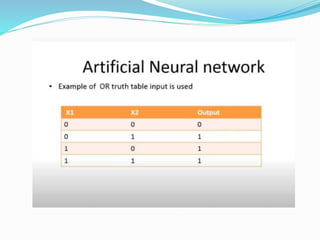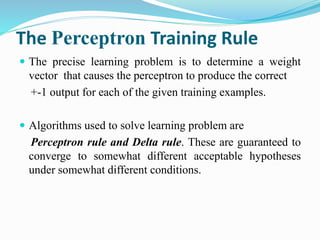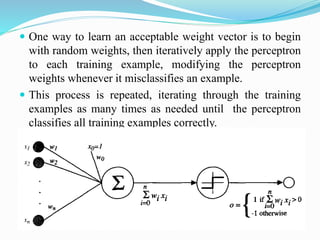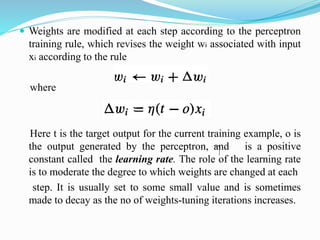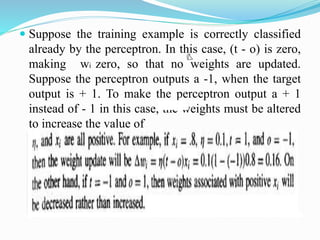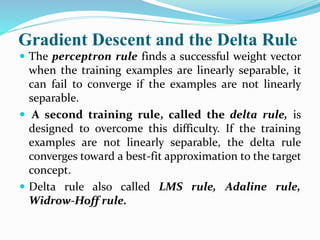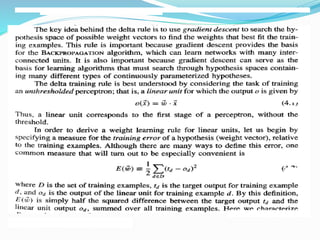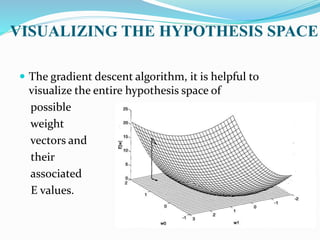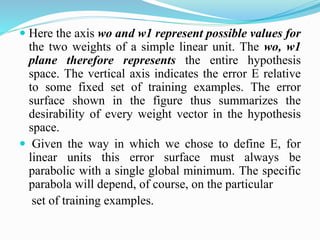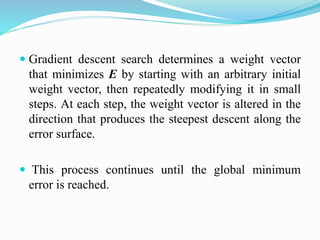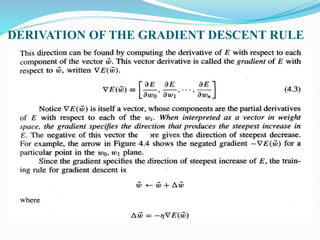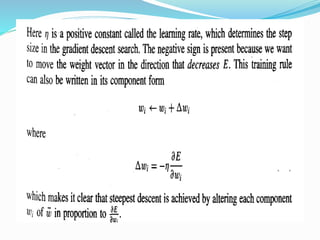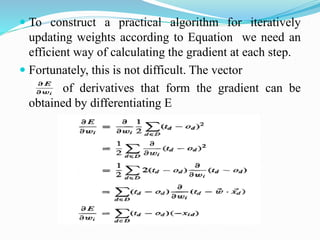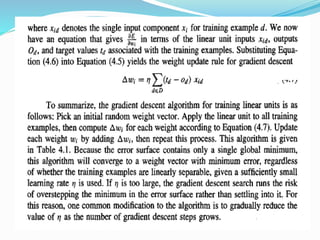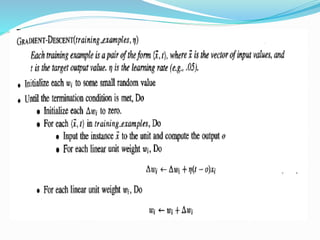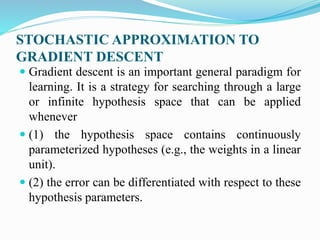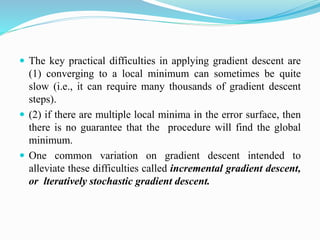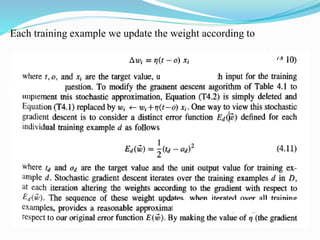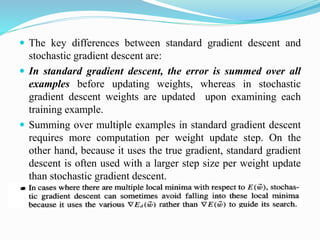1 of 40

### ML_Unit_2_Part_A

• 2. UNIT - II  Artificial Neural Networks-1:Introduction, neural network representation, appropriate problems for neural network learning, perceptions, multilayer networks and the back-propagation algorithm.  Artificial Neural Networks-2:Remarks on the Back- Propagation algorithm, An illustrative example: face recognition, advanced topics in artificial neural networks.  EvaluationHypotheses:Motivation,estimation hypothesis accuracy, basics of sampling theory, a general approach for deriving confidence intervals, difference in error of two hypotheses, comparing learning algorithms.
• 3. INTRODUCTION  Neural network learning methods provide a robust approach to approximating real-valued, discrete-valued, and vector-valued target functions.  Successful practical problems such as learning to recognize handwritten characters (LeCun et al. 1989), learning to recognize spoken words (Lang et al. 1990), and learning to recognize faces (Cottrell 1990).
• 4. BIOLOGICAL MOTIVATION  Artificial neural networks (ANNs) has been inspired in part by the observation that biological learning systems are built of very complex webs of interconnected neurons.  Artificial neural networks are built out of a densely interconnected set of simple units, where each unit takes a number of real-valued inputs (possibly the outputs of other units) and produces a single real-valued output (which may become the input to many other units).
• 5.  The human brain, for example, is estimated to contain a densely interconnected network of approximately 10^11 neurons, each connected, on average, to 10^4 others. Neuron activity is typically excited or inhibited through connections to other neurons.  The fastest neuron switching times are known to be on the order of 10^-3 seconds--quite slow compared to computer switching speeds of 10^-10 seconds.  One motivation for ANN systems is to capture this kind of highly parallel computation based on distributed representations.
• 6. • Historically, two groups of researchers have worked with artificial neural networks. • One group has been motivated by the goal of using ANNs to study and model biological learning processes. • A second group has been motivated by the goal of obtaining highly effective machine learning algorithms, independent of whether these algorithms mirror biological processes.
• 9. NEURAL NETWORK REPRESENTATIONS A prototypical example of ANN learning is provided by Pomerleau's (1993) system ALVINN, which uses a learned ANN to steer an autonomous vehicle driving at normal speeds on public highways. The input to the neural network is a 30 x 32 grid of pixel intensities obtained from a forward-pointed camera mounted on the vehicle. The network output is the direction in which the vehicle is steered. The ANN is trained to mimic the observed steering commands of a human driving the vehicle for approximately 5 minutes. ALVINN has used its learned networks to successfully drive at speeds up to 70 miles per hour and for distances of 90 miles on public highways
• 10.  ANN approaches, which are based on the BACKPROPAGATION algorithm .  The BACKPROPAGATION algorithm assumes the network is a fixed structure that corresponds to a directed graph, possibly containing cycles. Learning corresponds to choosing a weight value for each edge in the graph.  Although certain types of cycles are allowed, the vast majority of practical applications involve acyclic feed-forward networks, similar to the network structure used by ALVINN.
• 11. APPROPRIATE PROBLEMS FOR NEURAL NETWORK LEARNING  ANN learning is well-suited to problems in which the training data corresponds to noisy, complex sensor data, such as inputs from cameras and microphones.  The BACKPROPAGATION algorithm is the most commonly used ANN learning technique. It is appropriate for problems with the following characteristics:  Instances are represented by many attribute-value pairs:  In ALVINN example input attributes may be highly correlated or independent of one another. Input values can be any real values.  The target function output may be discrete-valued, real-valued, or a vector of several real- or discrete-valued attributes:  In the ALVINN system the output is a vector of 30 attributes, each corresponding to a recommendation regarding the steering direction.
• 12.  The training examples may contain errors. ANN learning methods are quite: robust to noise in the training data.  Long training times are acceptable: Network training algorithms typically require longer training times than, say, decision tree learning algorithms. Training times can range from a few seconds to many hours, depending on factors such as the number of weights in the network, the number of training examples considered, and the settings of various learning algorithm parameters.
• 13. Fast evaluation of the learned target function may be required:  Although ANN learning times are relatively long, evaluating the learned network, in order to apply it to a subsequent instance, is typically very fast. For example, ALVINN applies its neural network several times per second to continually update its steering command as the vehicle drives forward.  The ability of humans to understand the learned target function is not important:  The weights learned by neural networks are often difficult for humans to interpret. Learned neural networks are less easily communicated to humans than learned rules.
• 14.  The BACKPROPAGATION Algorithm for training multilayer networks of such units and consider several general issues such as the representational capabilities of ANNs, nature of the hypothesis space search, over fitting problems, and alternatives to the BACKPROPAGATION Algorithm.  A detailed example is also presented applying BACKPROPAGATION Algorithm recognition, and directions are provided for the reader to obtain the data and code to experiment further with this application.
• 15. PERCEPTRONS One type of ANN system is based on a unit called a perceptron. A perceptron takes a vector of real-valued inputs, calculates a linear combination of these inputs, then outputs a 1 if the result is greater than some threshold and -1 otherwise.
• 16.  More precisely, given inputs x1 through xn, the output o(x1, . . . , x,) computed by the perceptron is
• 17. Representational Power of Perceptions: We can view the perceptron as representing a hyperplane decision surface in the n-dimensional space of instances (i.e., points). The perceptron outputs a 1 for instances lying on one side of the hyperplane and outputs a -1 for instances lying on the other side. The equation for this decision Of course, some sets of positive and negative examples cannot be separated by any hyperplane. Those that can be separated are called linearly separable sets of examples.
• 18. ANN Single Layer Perceptron and Multi Layer Perceptron
• 19. Perceptron A single perceptron can be used to represent many boolean functions. For example, if we assume boolean values of 1 (true) and -1 (false), then one way to use a two-input perceptron to implement the AND function is to set the weights wo = -3, and wl = wz = .5. This perceptron can be made to represent the OR function instead by altering the threshold to wo = -.3. In fact, AND and OR can be viewed as special cases of m-of-n functions: that is, functions where at least m of the n inputs to the perceptron must be true. The OR function corresponds to rn = 1 and the AND function to m = n. Any m-of-n function is easily represented using a perceptron by setting all input weights to the same value (e.g., 0.5) and then setting the threshold wo accordingly.
• 20.  Perceptrons can represent all of the primitive boolean functions AND, OR, NAND (1 AND), and NOR (1 OR). Unfortunately, however, some boolean functions cannot be represented by a single perceptron, such as the XOR function whose value is 1 if and only if xl # xz.  The ability of perceptrons to represent AND, OR, NAND, and NOR is important because every boolean function can be represented by some network of interconnected units based on these primitives.
• 23. The Perceptron Training Rule  The precise learning problem is to determine a weight vector that causes the perceptron to produce the correct +-1 output for each of the given training examples.  Algorithms used to solve learning problem are Perceptron rule and Delta rule. These are guaranteed to converge to somewhat different acceptable hypotheses under somewhat different conditions.
• 24.  One way to learn an acceptable weight vector is to begin with random weights, then iteratively apply the perceptron to each training example, modifying the perceptron weights whenever it misclassifies an example.  This process is repeated, iterating through the training examples as many times as needed until the perceptron classifies all training examples correctly.
• 25.  Weights are modified at each step according to the perceptron training rule, which revises the weight wi associated with input xi according to the rule where Here t is the target output for the current training example, o is the output generated by the perceptron, and is a positive constant called the learning rate. The role of the learning rate is to moderate the degree to which weights are changed at each step. It is usually set to some small value and is sometimes made to decay as the no of weights-tuning iterations increases.
• 26.  Suppose the training example is correctly classified already by the perceptron. In this case, (t - o) is zero, making wi zero, so that no weights are updated. Suppose the perceptron outputs a -1, when the target output is + 1. To make the perceptron output a + 1 instead of - 1 in this case, the weights must be altered to increase the value of
• 27. Gradient Descent and the Delta Rule  The perceptron rule finds a successful weight vector when the training examples are linearly separable, it can fail to converge if the examples are not linearly separable.  A second training rule, called the delta rule, is designed to overcome this difficulty. If the training examples are not linearly separable, the delta rule converges toward a best-fit approximation to the target concept.  Delta rule also called LMS rule, Adaline rule, Widrow-Hoff rule.
• 29. VISUALIZING THE HYPOTHESIS SPACE  The gradient descent algorithm, it is helpful to visualize the entire hypothesis space of possible weight vectors and their associated E values.
• 30.  Here the axis wo and w1 represent possible values for the two weights of a simple linear unit. The wo, w1 plane therefore represents the entire hypothesis space. The vertical axis indicates the error E relative to some fixed set of training examples. The error surface shown in the figure thus summarizes the desirability of every weight vector in the hypothesis space.  Given the way in which we chose to define E, for linear units this error surface must always be parabolic with a single global minimum. The specific parabola will depend, of course, on the particular set of training examples.
• 31.  Gradient descent search determines a weight vector that minimizes E by starting with an arbitrary initial weight vector, then repeatedly modifying it in small steps. At each step, the weight vector is altered in the direction that produces the steepest descent along the error surface.  This process continues until the global minimum error is reached.
• 32. DERIVATION OF THE GRADIENT DESCENT RULE
• 34.  To construct a practical algorithm for iteratively updating weights according to Equation we need an efficient way of calculating the gradient at each step.  Fortunately, this is not difficult. The vector of derivatives that form the gradient can be obtained by differentiating E
• 37. STOCHASTIC APPROXIMATION TO GRADIENT DESCENT  Gradient descent is an important general paradigm for learning. It is a strategy for searching through a large or infinite hypothesis space that can be applied whenever  (1) the hypothesis space contains continuously parameterized hypotheses (e.g., the weights in a linear unit).  (2) the error can be differentiated with respect to these hypothesis parameters.
• 38.  The key practical difficulties in applying gradient descent are (1) converging to a local minimum can sometimes be quite slow (i.e., it can require many thousands of gradient descent steps).  (2) if there are multiple local minima in the error surface, then there is no guarantee that the procedure will find the global minimum.  One common variation on gradient descent intended to alleviate these difficulties called incremental gradient descent, or lteratively stochastic gradient descent.
• 39. Each training example we update the weight according to
• 40.  The key differences between standard gradient descent and stochastic gradient descent are:  In standard gradient descent, the error is summed over all examples before updating weights, whereas in stochastic gradient descent weights are updated upon examining each training example.  Summing over multiple examples in standard gradient descent requires more computation per weight update step. On the other hand, because it uses the true gradient, standard gradient descent is often used with a larger step size per weight update than stochastic gradient descent. 